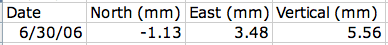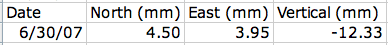# Part 2—Explore GPS Data

## Step 1 – Learn What the Values Mean

1. Take a look at the data at the top of the spreadsheet from Part 1. The first eight rows of the file contain information about the SEAT GPS station.
2. Row 8 shows the Reference Position of the station: this is the zero point from which initial GPS positions were measured. The latitude, longitude, and elevation reported for the Reference Position describe the location of the single point where the unit was first installed.
3. Line 9 shows the column headings for the GPS position data.
4. Below row 9, the first column of data contains the dates for which GPS positions were collected.
5. Values in the columns labeled North (mm), East (mm), and Vertical (mm) represent the daily location of the station with respect to a reference point. All three measurements are necessary to characterize the position of the GPS unit in three dimensions.
• The North column represents the distance (in mm) between the unit and the zero point in a North-South direction. Positive values indicate distances north of the zero point; negative values indicate distances south of the zero point.
• The East column shows how far East (+) or West (-) of the zero position the unit is.
• The Vertical column shows how far above (+) or below (-) the zero point the unit is.

6. The remaining columns show the standard deviation of the data values in each direction and a qualifier about the data quality. This activity does not address these concepts.
Daily Positions

The values given in the spreadsheet are daily positions for each GPS unit. These positions are 24-hour averages of location data collected every 15 seconds. Averaging removes position errors due to processes such as electronic noise in the receiver, deviations in expected satellite positions, and reflection and atmospheric delay of the GPS signals.

## Step 2-Practice Interpreting the Data

1. Consider the meaning of this data record. Describe what it tells about the SEAT GPS unit.2. Check these data to interpret the location of the SEAT GPS unit one year later.3. Scan down the values in the columns of North and East position data. You'll see that the position of the GPS station changes slightly every day. Over longer periods, from the beginning of the records to the end, for instance, a general trend in the position of the GPS unit becomes obvious.